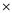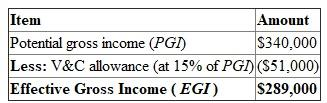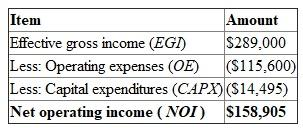# Quiz 18: Investment Decisions: Ratios

Business

Effective Gross Income (EGI) : Effective Gross Income is calculated by deducting the Vacancy and collection (V C) loss from Gross Potential Income (GPI). First year gross potential income (PGI) is \$340,000 Vacancy and collection (V C) loss is 15% of gross potential income Therefore, (V C) allowance = [\$340,00015%] = \$51,000 Calculate Effective Gross Income (EGI) for the first year of operations:Hence the EGI is \$289,000.

We are asked to choose the appropriate solution from the given options to complete the given statement. Income multipliers : The correct answer is option A. are useful as a preliminary analysis tool to weed out obviously unacceptable investment opportunities. Income multiplier analysis is an old, simple analysis tool that shows the relationship between price and either gross or net income. Multipliers are not adequate tools of analysis by themselves, but the tool helps analysts to quickly and inexpensively weed out obviously unacceptable investments.

Effective Gross Income (EGI) : Effective Gross Income is calculated by deducting the Vacancy and collection (V C) loss from Gross Potential Income (GPI). First year gross potential income (PGI) is \$340,000 Vacancy and collection (V C) loss is 15% of gross potential income Therefore, (V C) allowance = [\$340,00015%] = \$51,000 Calculate Effective Gross Income (EGI) for the first year of operations:Hence the EGI is \$289,000. Net Operating Income (NOI) is calculated by deducting the operating expenses and capital expenditure from Effective Gross Income (EGI). Operating expenses are equal to 40% of EGI Capital expenditure is equal to 5% of EGI Effective Gross Income (EGI) = \$289,000 Operating expenses = [\$289,00040%] = \$115,600 Capital expenditure = [\$289,0005%] = \$14,495 Calculate Net Operating Income (NOI) for the first year of operations :Hence the NOI is \$158,905.